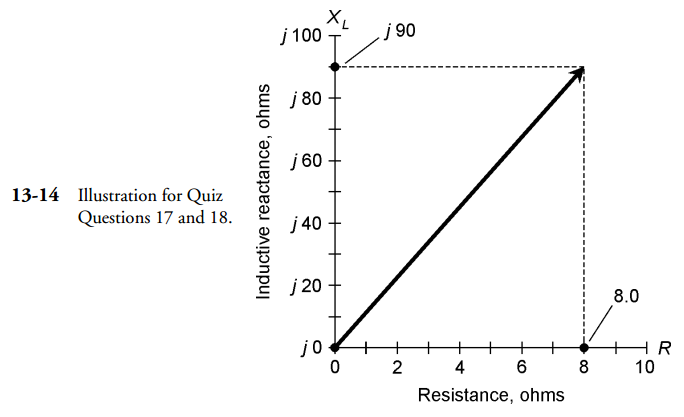# Gibilisco: MCQ in Inductive Reactance | ECE Board Exam

(Last Updated On: April 4, 2022)This is the Multiple Choice Questions (MCQs) in Chapter 13: Inductive Reactance from the book Teach Yourself Electricity and Electronics, 5th edition by Stan Gibilisco. If you are looking for a reviewer in Electronics Engineering this will definitely help you before taking the Board Exam.

Start Practice Exam Test Questions

Choose the letter of the best answer in each questions.

1. As the number of turns in a coil increases, the current in the coil will eventually:

A. Become very large.

B. Stay the same.

C. Decrease to near zero.

D. Be stored in the core material.

Solution:

2. As the number of turns in a coil increases, the reactance:

A. Increases.

B. Decreases.

C. Stays the same.

D. Is stored in the core material.

Solution:

3. As the frequency of an ac wave gets lower, the value of XL for a particular coil:

A. Increases.

B. Decreases.

C. Stays the same.

D. Depends on the voltage.

Solution:

4. A coil has an inductance of 100 mH. What is the reactance at a frequency of 1000 Hz?

A. 0.628 Ω.

B. 6.28 Ω.

C. 62.8 Ω.

D. 628 Ω.

Solution:

5. A coil shows an inductive reactance of 200 Ω at 500 Hz. What is its inductance?

A. 0.637 H.

B. 628 H.

C. 63.7 mH.

D. 628 mH.

Solution:

6. A coil has an inductance of 400 μH. Its reactance is 33 Ω. What is the frequency?

A. 13 kHz.

B. 0.013 kHz.

C. 83 kHz.

D. 83 MHz.

Solution:

7. An inductor has XL = 555 Ω at f = 132 kHz. What is L?

A. 670 mH.

B. 670 μH.

C. 460 mH.

D. 460 μH.

Solution:

8. A coil has L = 689 μH at f =990 kHz. What is XL?

A. 682 Ω.

B. 4.28 Ω.

C. 4.28 K Ω.

D. 4.28 M Ω.

Solution:

9. An inductor has L = 88 mH with XL = 100 Ω. What is f?

A. 55.3 kHz.

B. 55.3 Hz.

C. 181 kHz.

D. 181 Hz.

Solution:

10. Each point in the RL plane:

A. Corresponds to a unique resistance.

B. Corresponds to a unique inductance.

C. Corresponds to a unique combination of resistance and inductive reactance.

D. Corresponds to a unique combination of resistance and inductance.

Solution:

11. If the resistance R and the inductive reactance XL both vary from zero to unlimited values, but are always in the ratio 3:1, the points in the RL plane for all the resulting impedances will fall along:

A. A vector pointing straight up.

B. A vector pointing “east.”

C. A circle.

D. A ray of unlimited length.

Solution:

12. Each impedance R + jXL:

A. Corresponds to a unique point in the RL plane.

B. Corresponds to a unique inductive reactance.

C. Corresponds to a unique resistance.

D. All of the above.

Solution:

13. A vector is a quantity that has:

A. Magnitude and direction.

B. Resistance and inductance.

C. Resistance and reactance.

D. Inductance and reactance.

Solution:

14. In an RL circuit, as the ratio of inductive reactance to resistance, XL/R, decreases, the phase angle:

A. Increases.

B. Decreases.

C. Stays the same.

D. Cannot be found.

Solution:

15. In a purely reactive circuit, the phase angle is:

A. Increasing.

B. Decreasing.

C. 0 degrees.

D. 90 degrees.

Solution:

16. If the inductive reactance is the same as the resistance in an RL circuit, the phase angle is:

A. 0 degrees.

B. 45 degrees.

C. 90 degrees.

Solution:

D. Impossible to find; there’s not enough data given.

NOTE: For questions 17 – 18 see Fig.13-14.17. In Fig. 13-14, the impedance shown is:

A. 8.0.

B. 90.

C. 90 + j8.0.

D. 8.0 + j90.

Solution:

18. In Fig. 13-14, note that the R and XL scale divisions are of different sizes. The phase angle is:

A. About 50 degrees, from the looks of it.

B. 48 degrees, as measured with a protractor.

C. 85 degrees, as calculated trigonometrically.

D. 6.5 degrees, as calculated trigonometrically.

Solution:

19 An RL circuit consists of a 100-μH inductor and a 100-Ω resistor. What is the phase angle at a frequency of 200 kHz?

A. 45.0 degrees.

B. 51.5 degrees.

C. 38.5 degrees.

D. There isn’t enough data to know.

Solution:

20. An RL circuit has an inductance of 88 mH. The resistance is 95 Ω. What is the phase angle at 800 Hz?

A. 78 degrees.

B. 12 degrees.

C. 43 degrees.

D. 47 degrees.

Solution:

### Topics Included in the Test from Chapter 9 to Chapter 18

Part 2: Alternating Current
Chapter 10: MCQ in Inductance
Chapter 11: MCQ in Capacitance
Chapter 12: MCQ in Phase

### Complete List of Multiple Choice Questions from this Book

PinoyBIX Engineering. © 2014-2021 All Rights Reserved | How to Donate? |